# HRC-S High Voltage Test Analysis Plots

## INDEX

Count rate increases with voltage
Average background subtracted count rate for the zeroth order for each ObsID. The black line is for unfiltered evt1 and the red line is for status-bit filtered and properly time-filtered events. The error bars are shown as vertical lines at each point. The ObsIDs corresponding to each point are labeled. The horizontal dotted line shows the initial count rate level before the test sequence. As the voltage is increased, the overall count rate also increases. After the test, the count rate returns to the original level.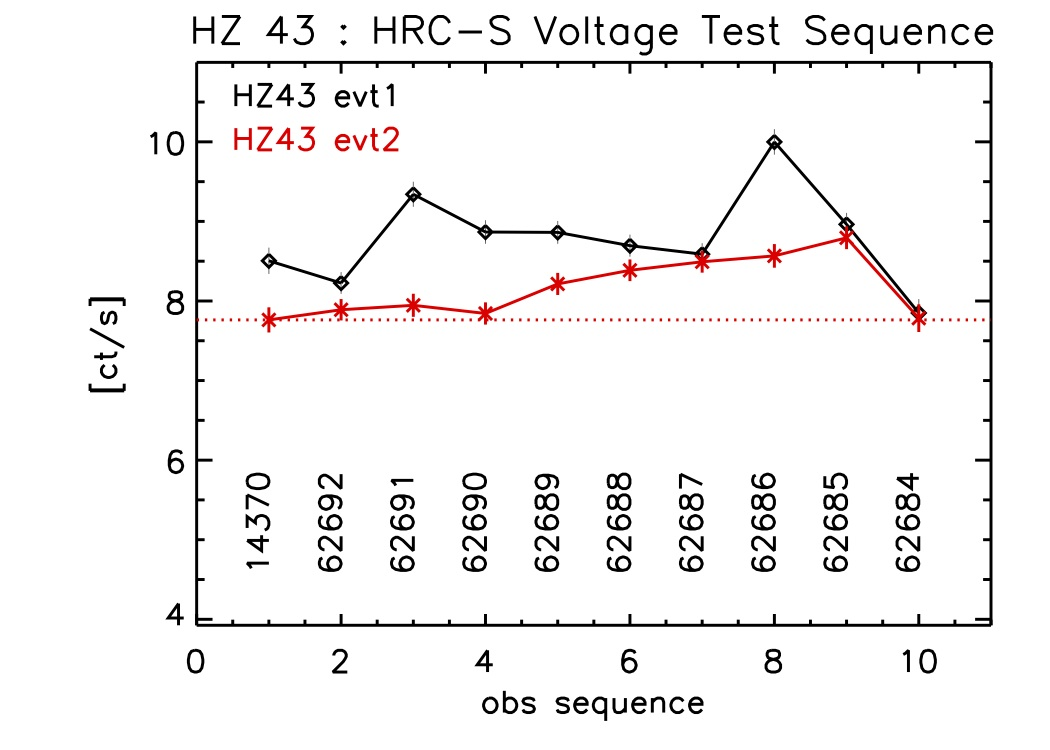[.pdf]
Background subtracted count rate for the dispersed events for each ObsID. The solid red line is the count rate for events in the bowtie background region (not scaled to the source area). 1-sigma error bars are shown for all points, and are color coded by the ObsID (listed along the bottom of the plot) for the source rates. The red dotted line marks the value of the count rate in the first observation. Note that the background rate dips as the voltage is increased.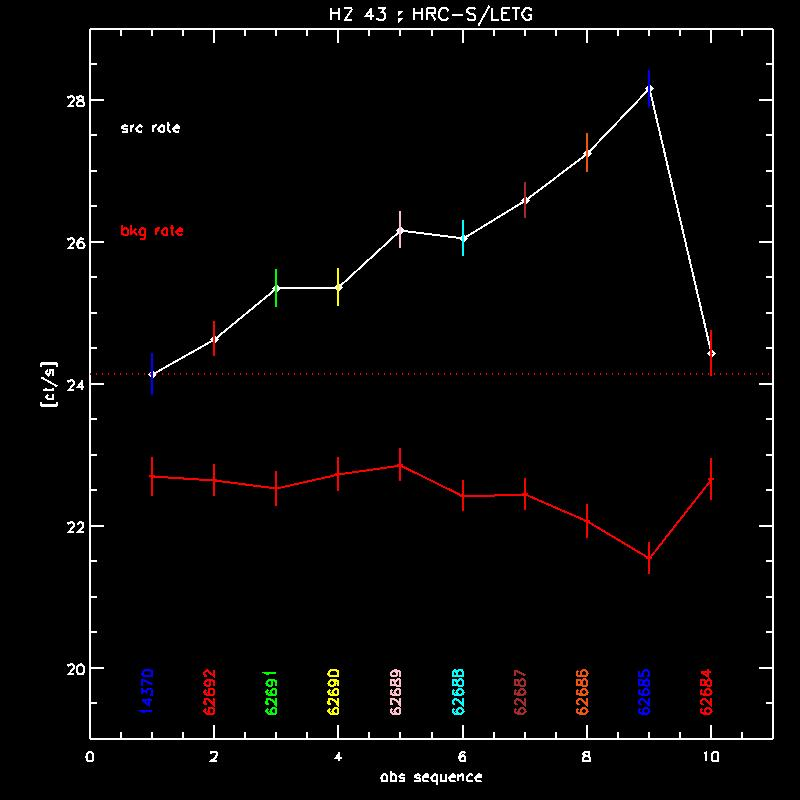[.pdf]
Running average background subtracted count rate for each ObsID. Each ObsID is coded by one color, and the actual observation time is shown along the X-axis. The running average is taken over 500 photons, so the statistical uncertainty is approx 4.4%. The actual variation is much higher (>~10%), and is attributable to QE variations along the dither path. (See below for a graphic illustration.)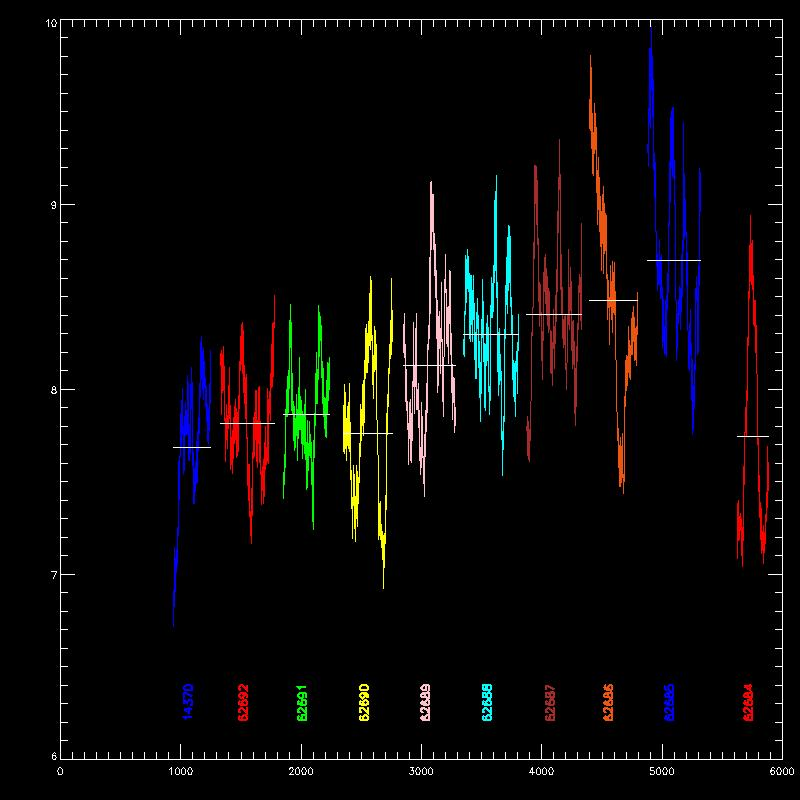[.pdf]

Changes in PHA and PI with time
Scatter plots of PHA and PI vs time for all the ObsIDs. The last observation (62684) which has the same voltage settings as the first one (14370), is also shown folded back and displayed next to the first one (but in a different color) to facilitate easy comparison. The mean PHA and PI (shown as running means; red curves) increase for each observation, as expected. Also shown are the running 16th and 84th percentiles (green curves), which show the spectrum getting wider in absolute terms as the voltage increases. There is also considerable jitter in the mean PHAs, which are mostly due to QE variations along the dither path, and which are mostly (but not completely) removed by the gain corrections.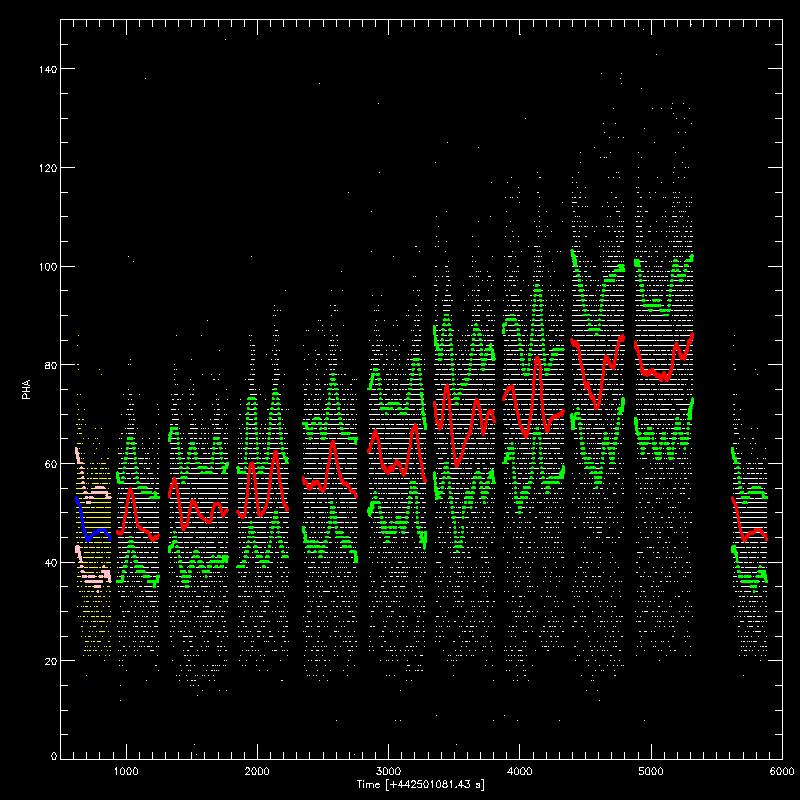[.pdf]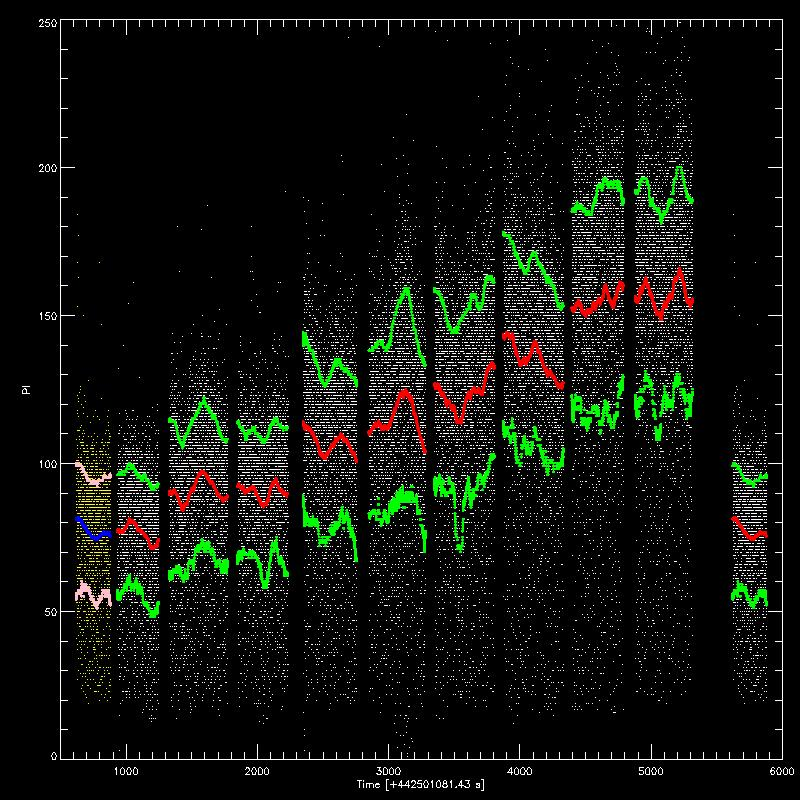[.pdf]

PHA and PI vs count rate
Scatter plots of running mean PHA (X-axis) vs running mean count rates (Y-axis, left plot), where the mean is computed for 500 events at a time. Data from each ObsID is coded separately with a different color (but watch out for two duplicates). The absolute count rates show an increase as the mean PHA increases, as expected. The initial impression that there is a linear trend even within each observation is dispelled by looking at the adjacent plots, where the normalized running mean count rates are plotted along the Y-axis. The normalization is computed as (<ctrt>-average), where average is the averate count rate for the entire ObsID.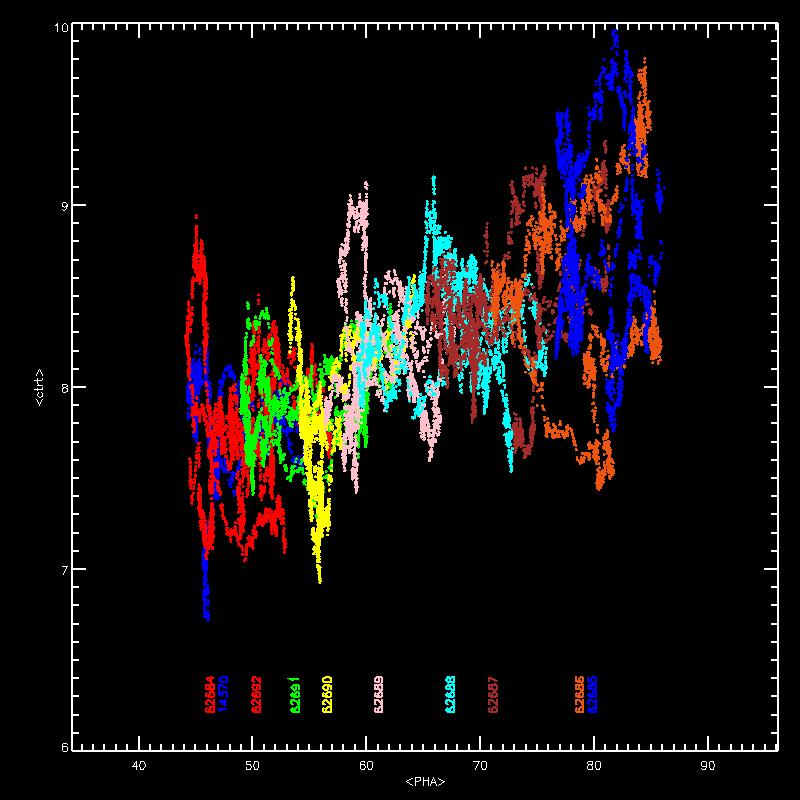[.pdf]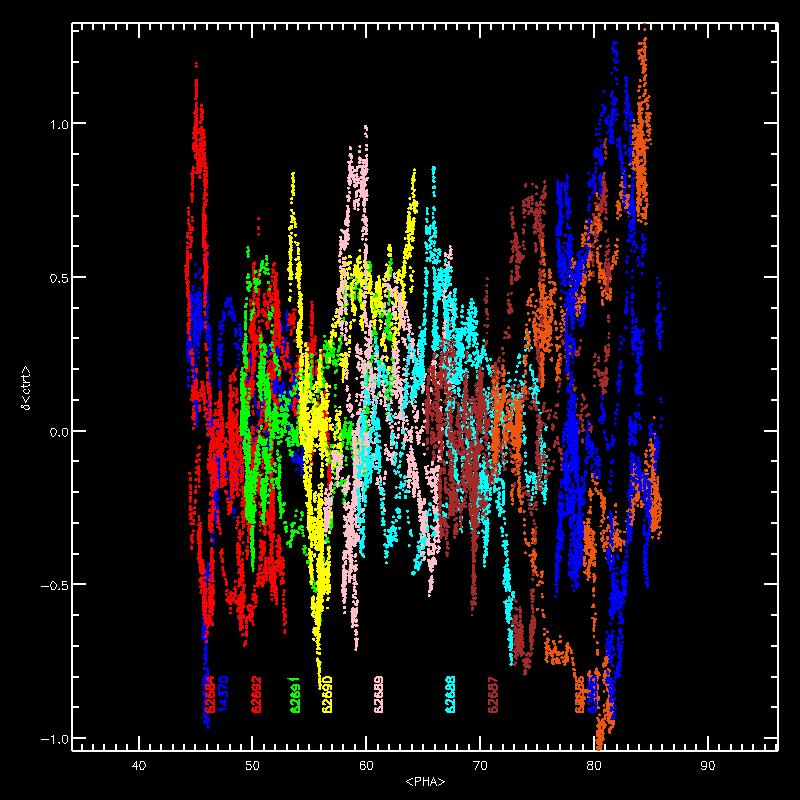[.pdf]
Same as above, for running mean PI instead.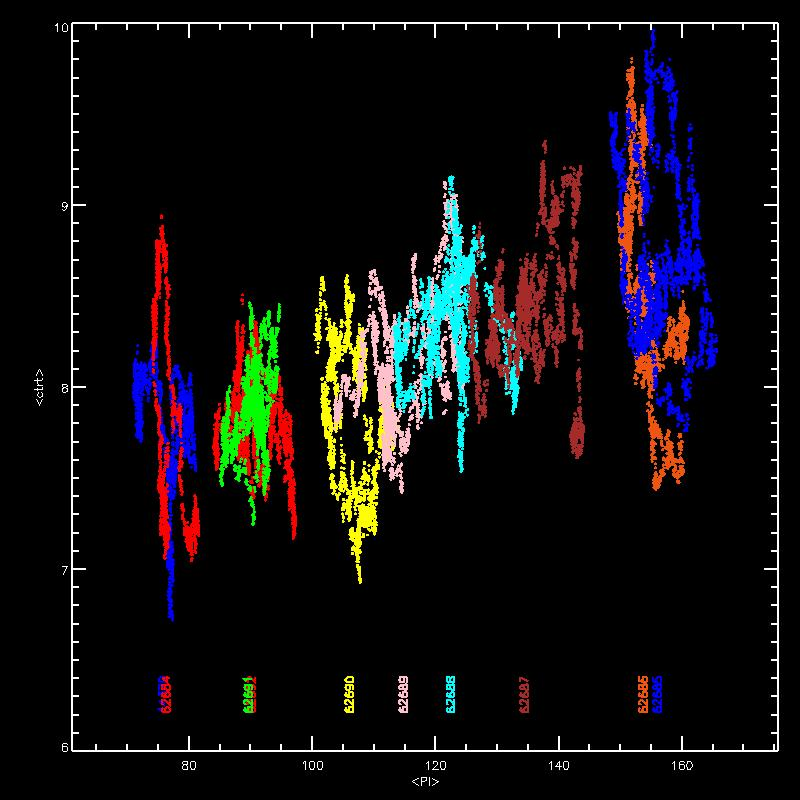[.pdf]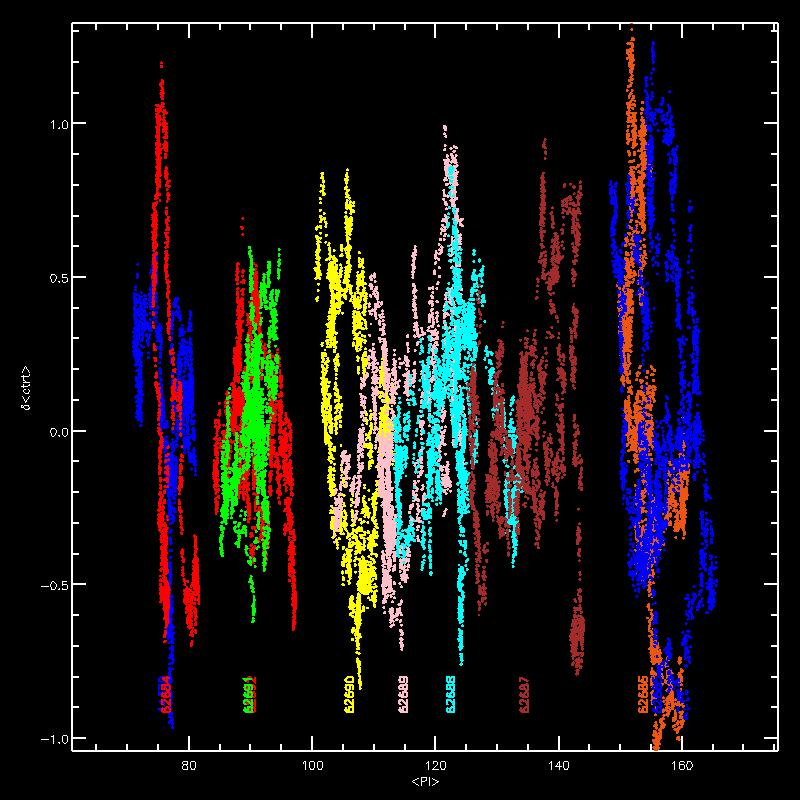[.pdf]
For dispersed spectra, changes in count rates as a function of wavelength, relative to the first observation. The summed 1st order rate ratios are shown as the central group of curves, color coded according to ObsID, and the +1 and -1 order spectra are shown separately, offset by +0.5 and -0.5 for clarity. The spectra are binned over 4, 8, and 16 Angstrom wide bins. The counts spectra from the first observation is also shown at the bottom, scaled to a maximum of 1/3 for all (dot-dashed), +ve (dashed), and -ve (dotted).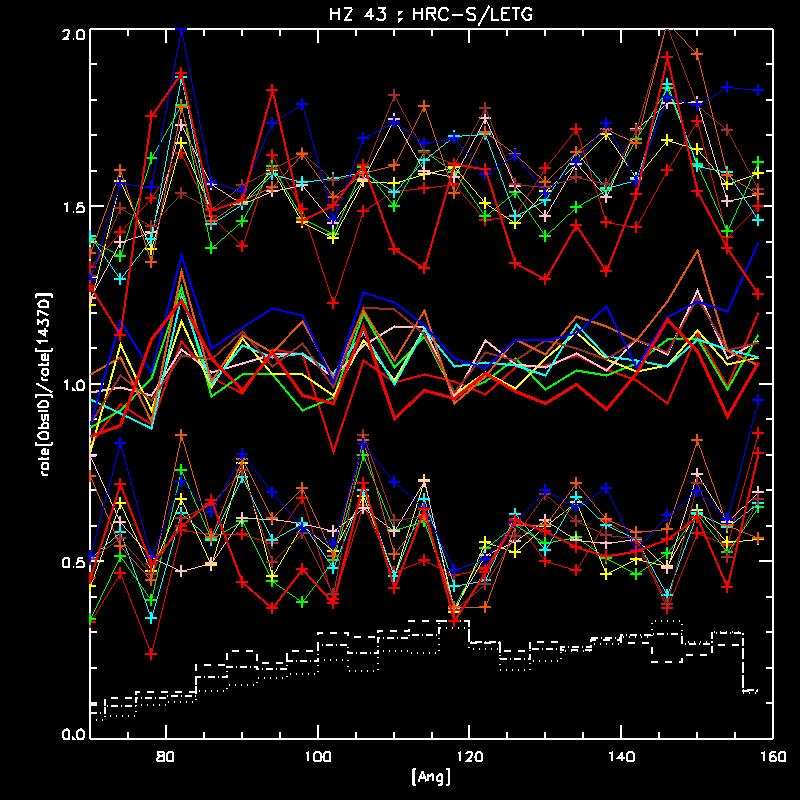[.pdf]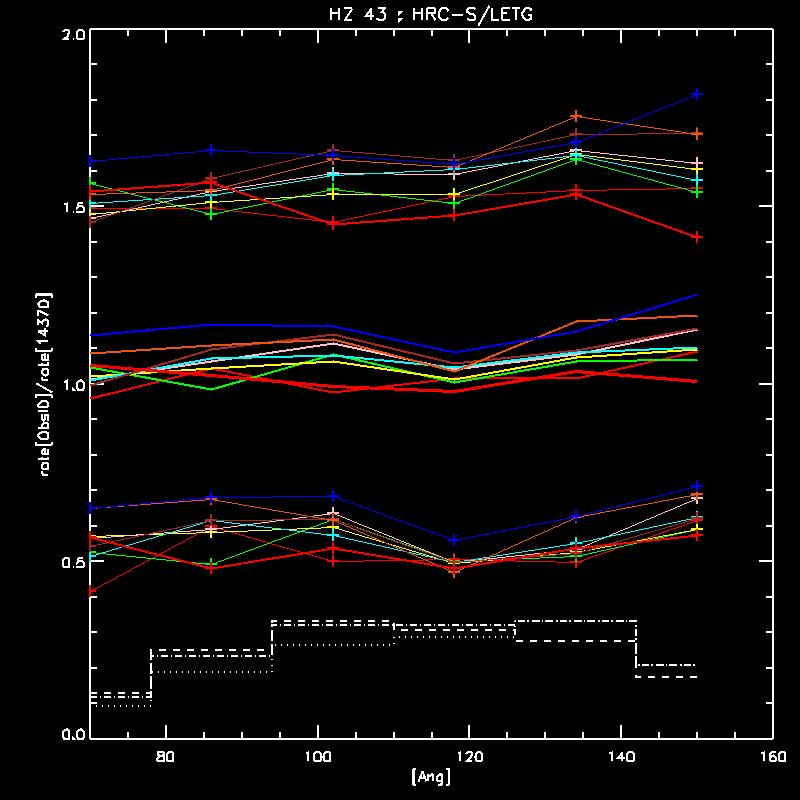[.pdf]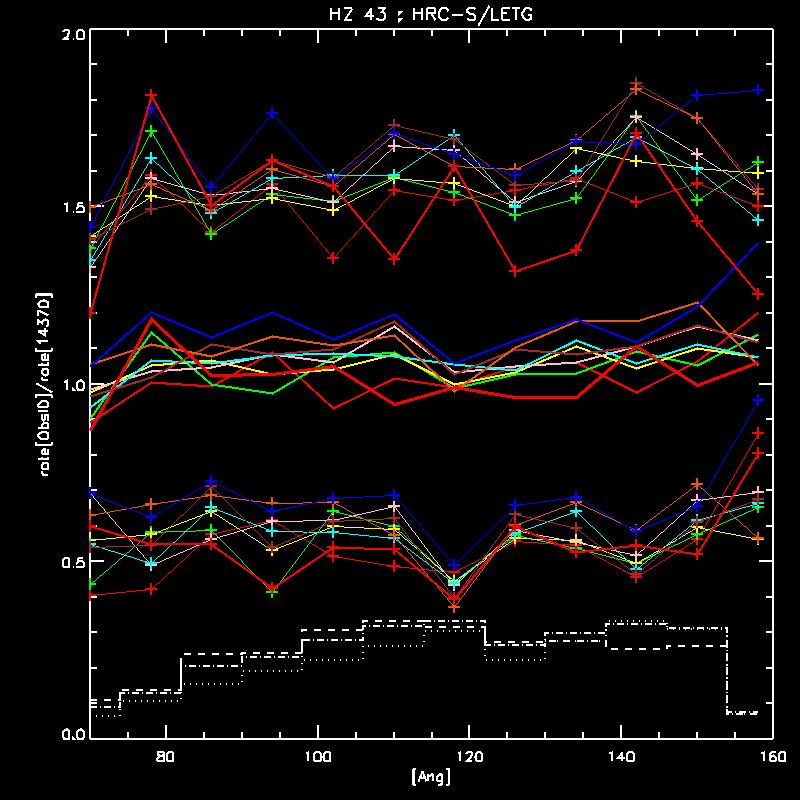[.pdf]

DET vs count rate
Much of the variations seen in PHA, PI, and count rates within an ObsID in the above plots can be attributed to QE variations along the dither path, as is illustrated below. In the first plot, a scatter plot of DETY vs DETX is shown, with the events color coded according to ObsID (same color scheme as above). The observations start with the blue swoosh going center/right to top/left, and follows on with red, green, yellow, pink, cyan, brown, orange, blue, and red, ending slightly upwards of the center of the pattern. The next plot shows the same pattern, but the colors are now coded by absolute count rates, with darker shades representing higher rates. The third plot shows the count rates normalized separately for each ObsID (as <ctrt>-average, see above), with an additional visual aid of point size depicting the magnitude. The fourth plot is the same as the third, where point-size depicts the normalized count rate, but the colors are now coded according to ObsID. There is some indication that QE variations do not completely account for the count rate variations, but establishing this requires more work.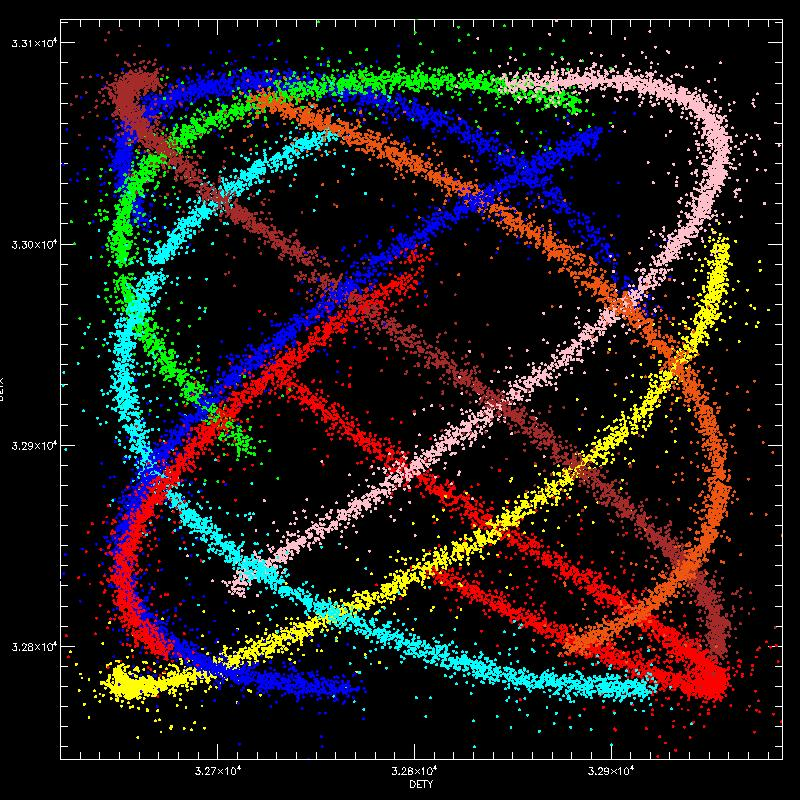[.pdf]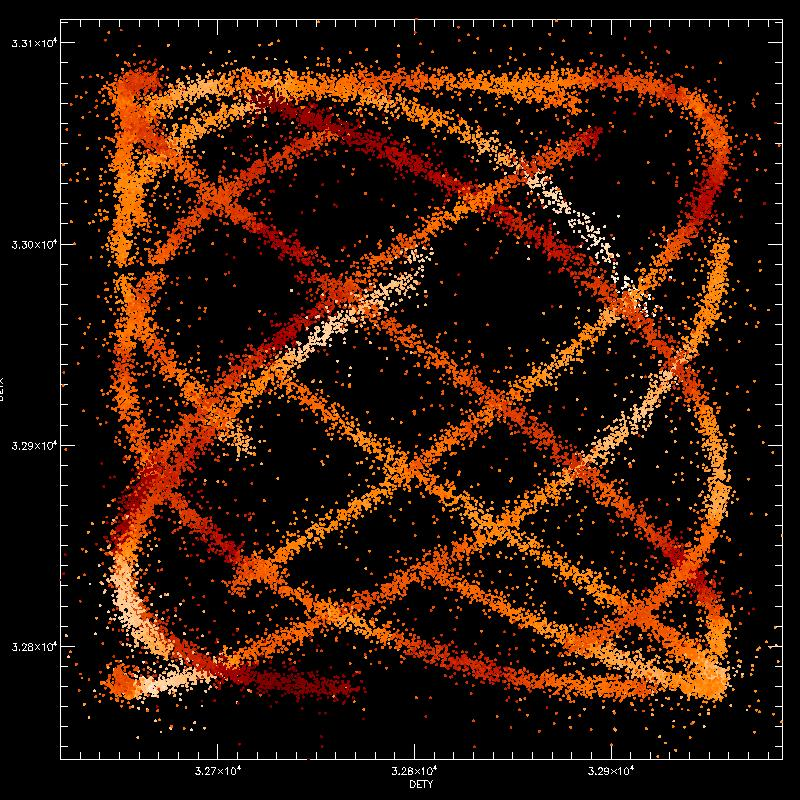[.pdf]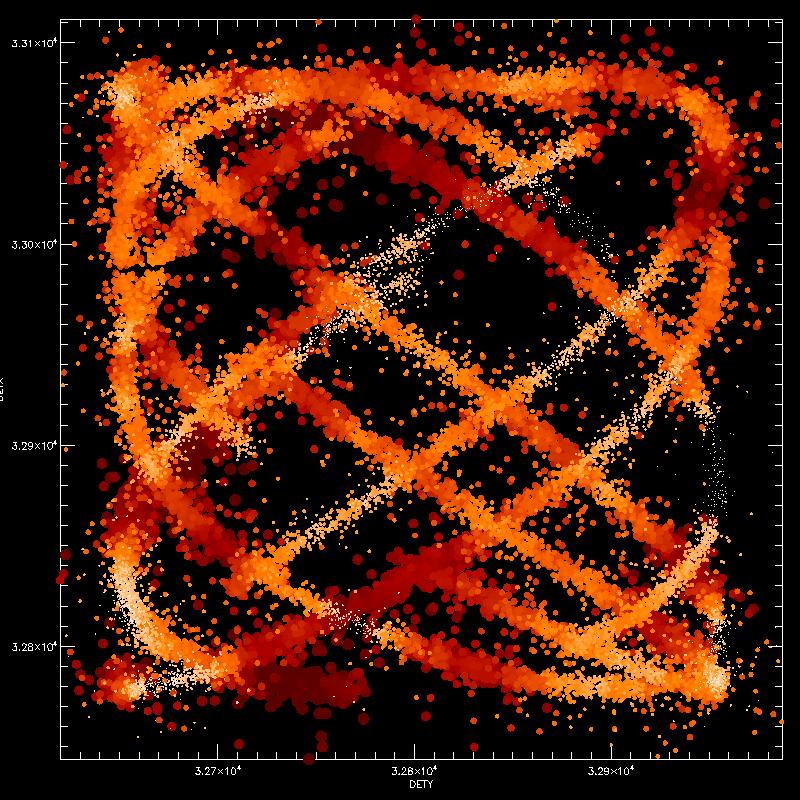[.pdf]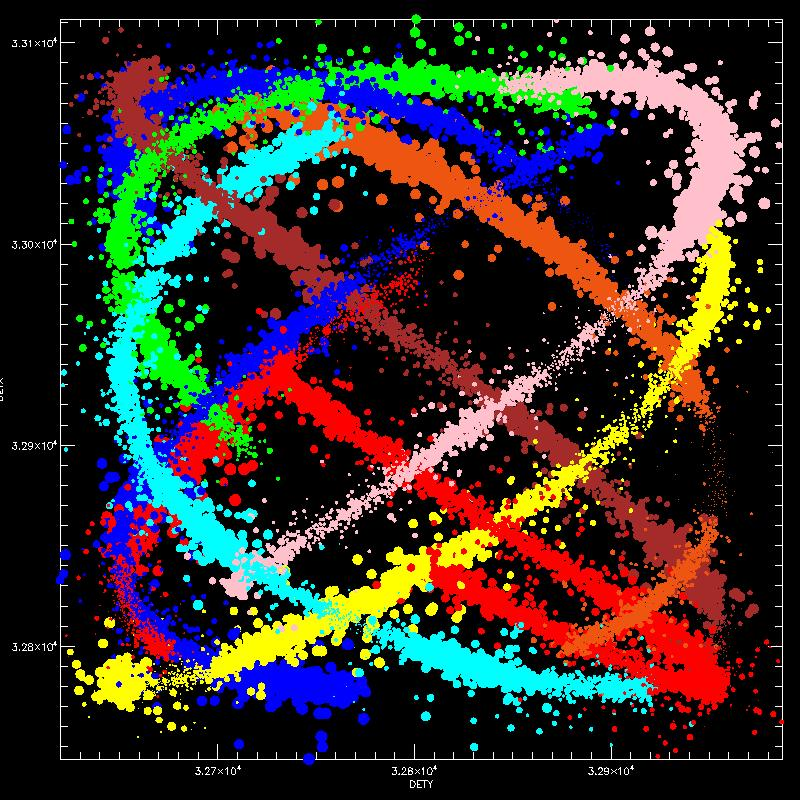[.pdf]
For dispersed spectra, changes in count rates as a function of DETX, relative to the first observation. The curves are color coded according to ObsID. The spectra are binned over 500 pixel wide bins. The counts spectrum from the first observation is also shown at the bottom, scaled to a maximum of 1.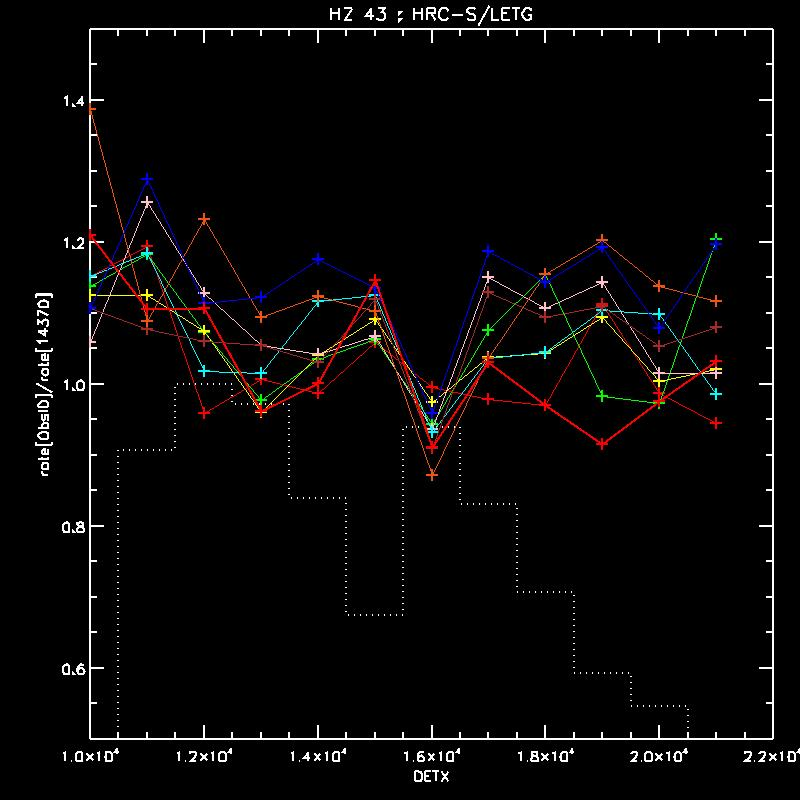[.pdf]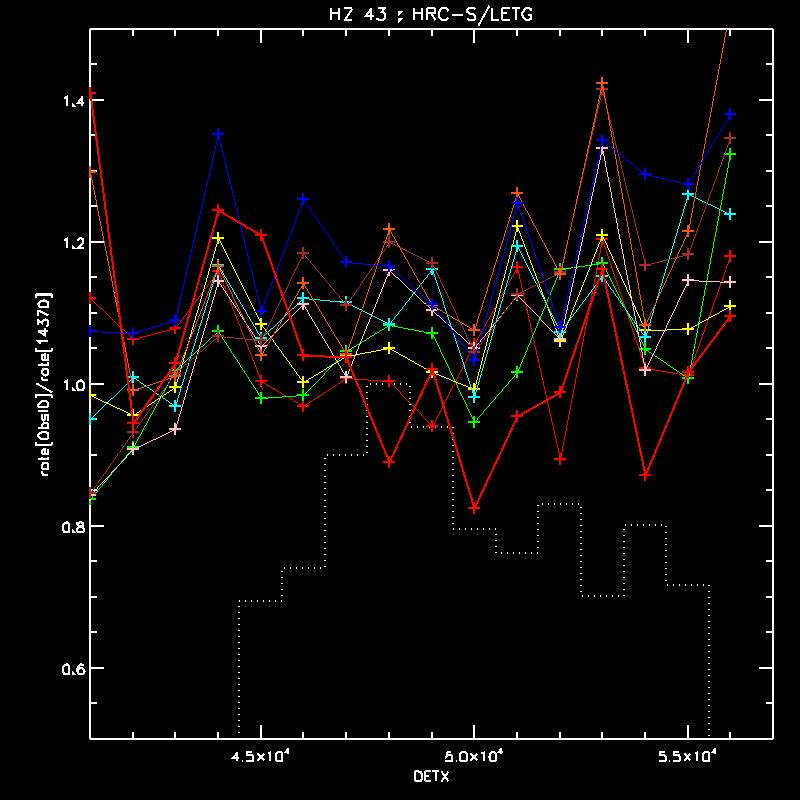[.pdf]